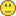# QlikView App Dev

Discussion Board for collaboration related to QlikView App Development.

Announcements
Now Live: Qlik Sense SaaS Simplified Authoring – Analytics Creation for Everyone: READ DETAILS
cancel
Showing results for
Did you mean:Specialist

## How to solve this using IF Statement

I have a problem that might be fixed by a proper IF statement. Hoping you experts might be able to help me out.

Problem:

I have 3 products A B & C. During month(now) which is april, I want to forecast my sales for B&C but not A. for the past months, there is no need to forecast so you will see actual sales volume same as forecasted sales volume in the sample table.

What I have so far:

To make the espression work on front end with ease, I created a flag field in the script:- if (monthstart([Sales Month]) = monthstart(today()) and Product <> ‘A’, ‘Flag’, ‘No_Forcast’) as Flag_Field

I am using this calculated field in the expression in the bar chart.
if(Flag_Field='Flag',(sum([Actual SalesVolume])/VNo_days)*VTotal_days,

sum([ActualSales Volume])

but the problem is unless I select a single product, either A, B, or C the month(now) metric value does not show correct result. If I select A and B, the metric shown is the actual volume, it does not take into account the forecasted volume.

Problem defined againwhen product field is not selected, Result shown = (20000+8000+3000) = 31000. Result expected =(20000+40000+15000) = 75000

when a single product is selected, for eg B, it will correctly show 40000shout out to stalwar1‌ sunny you dont have to solve this if it requires hard workbut just wanted to thank you for your help in the past. best.

1 Solution

Accepted SolutionsMVP

RangeSum(

Sum({<Flag_Field = {'Flag'}>}[Actual SalesVolume])/VNo_days)*VTotal_days,

Sum({<Flag_Field = {'No_Forcast'}>} ActualSales Volume]))

4 RepliesMVP

RangeSum(

Sum({<Flag_Field = {'Flag'}>}[Actual SalesVolume])/VNo_days)*VTotal_days,

Sum({<Flag_Field = {'No_Forcast'}>} ActualSales Volume]))Specialist
Author

Its actually a ratio that I am measuring. so in the previous formual i added /sum(Totalcost) to measure a ratio which will make the expression as below. and rangesum do not seem to work.

if(Flag_Field='Flag',((sum([Actual SalesVolume])/VNo_days)*VTotal_days)/sum(Totalcost),

sum([ActualSales Volume]/sum(Totalcost)

I left the problem to have only sum to simplify the problem, now I may have made it complex. sorry about that.MVP

May be just this

RangeSum(

Sum({<Flag_Field = {'Flag'}>}[Actual SalesVolume])/VNo_days)*VTotal_days,

Sum({<Flag_Field = {'No_Forcast'}>} ActualSales Volume]))

/

Sum([Totalcost])Specialist
Author

My apologies Sunny. you solved it the first time as always with your midas touch man. I was not thinking straight and missed a bracketThank you so much. have a wonderful weekend.